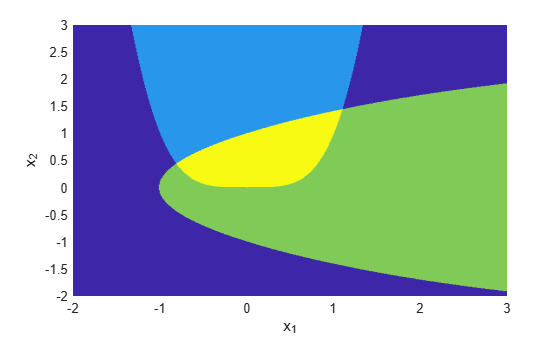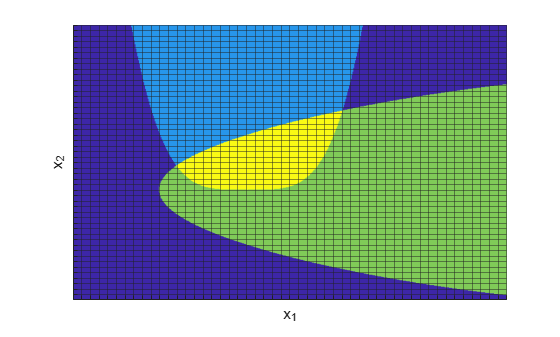# Solve Nonlinear Problem with Integer and Nonlinear Constraints

The `surrogateopt` solver accepts both integer constraints and nonlinear constraints. Compare the solution of a nonlinear problem both with and without integer constraints. The integer constraints cause the solution to lie on a reasonably fine grid.

### Objective and Constraint Functions

The objective function is

`$f\left(x\right)=\mathrm{log}\left(1+3{\left({x}_{2}-\left({x}_{1}^{3}-{x}_{1}\right)\right)}^{2}+\left({x}_{1}-4/3{\right)}^{2}\right).$`

This objective function is nonnegative, and takes its minimum value of 0 at the point $x=\left[4/3,\left(4/3{\right)}^{3}-4/3\right]$ = [1.3333, 1.0370].

The problem has two nonlinear constraint functions.

`$\begin{array}{l}{x}_{1}^{4}\le 5\mathrm{sinh}\left({x}_{2}/5\right),\\ {x}_{2}^{2}\le 5\mathrm{tanh}\left({x}_{1}/5\right)+1.\end{array}$`

Plot the feasible region for the nonlinear constraints.

```[X,Y] = meshgrid(-2:.01:3); Z = (5*sinh(Y./5) >= X.^4); % Z=1 where the first constraint is satisfied, Z=0 otherwise Z = Z+ 2*(5*tanh(X./5) >= Y.^2 - 1); % Z=2 where the second constraint is satisfied % Z=3 where both constraints are satisfied surf(X,Y,Z,'LineStyle','none'); fig = gcf; fig.Color = 'w'; % white background view(0,90) xlabel('x_1') ylabel('x_2')```The yellow region shows where both constraints are satisfied.

`surrogateopt` requires that the objective and constraint functions are part of the same function, one that returns a structure. The objective function is in the `Fval` field of the structure, and the constraints are in the `Ineq` field. These fields are the output of the `objconstr` function at the end of this example.

### Scale Integer Constraints to Lie on Fine Grid

Set the problem to have integer constraints in both variables, `x(1)` and `x(2)`.

`intcon = [1 2];`

Scale the problem so that the variables are scaled by `s = 1/10`, where `s` multiplies the variables.

```s = 0.1; f = @(x)objconstr(x,s);```

For this scaling to be effective, you need to scale the bounds by $1/s$. Set the unscaled bounds to $-2\le {x}_{i}\le 3$ and scale each by $1/s$.

```lb = [-2,-2]/s; ub = [3,3]/s;```

By using the scaling `s`, the problem effectively has spacing of `s` in each component `x(1)` and `x(2)`. Plot the integer points as a grid with spacing `s`.

```hold on grid on ax = gca; sp = -2:s:3; ax.XTick = sp; ax.YTick = sp; ax.Layer = 'top'; ax.GridAlpha = 1/2; ax.XTickLabel = ''; ax.YTickLabel = ''; xlabel('x_1') ylabel('x_2') hold off```### Solve Scaled Problem

Set options to use tighter constraints than the default, and to use the `surrogateoptplot` plot function.

`opts = optimoptions('surrogateopt','PlotFcn',"surrogateoptplot","ConstraintTolerance",1e-6);`

Call `surrogateopt` to solve the problem.

```rng default % For reproducibility [sol,fval,eflag,outpt] = surrogateopt(f,lb,ub,intcon,opts)``````surrogateopt stopped because it exceeded the function evaluation limit set by 'options.MaxFunctionEvaluations'. ```
```sol = 1×2 5 1 ```
```fval = 0.8634 ```
```eflag = 0 ```
```outpt = struct with fields: elapsedtime: 69.0582 funccount: 200 constrviolation: -0.0375 ineq: [-0.0375 -1.4883] rngstate: [1x1 struct] message: 'surrogateopt stopped because it exceeded the function evaluation limit set by ...' ```

Plot the solution as a red circle on the figure. Notice that the objective function value is approximately 0.86.

```figure(fig); hold on plot3(sol(1)*s,sol(2)*s,5,'ro') hold off```### Compare to Solution Without Integer Constraints

Compare the solution with integer constraints to the solution without integer constraints.

`[sol2,fval2,eflag2,outpt2] = surrogateopt(f,lb,ub,[],opts)````surrogateopt stopped because it exceeded the function evaluation limit set by 'options.MaxFunctionEvaluations'. ```
```sol2 = 1×2 4.3631 0.3626 ```
```fval2 = 0.8153 ```
```eflag2 = 0 ```
```outpt2 = struct with fields: elapsedtime: 48.0638 funccount: 200 constrviolation: -1.7294e-05 ineq: [-1.7294e-05 -1.4339] rngstate: [1x1 struct] message: 'surrogateopt stopped because it exceeded the function evaluation limit set by ...' ```

Here, the objective function value is approximately 0.815. The integer constraints increase the objective function value by less than 10%. Plot the new solution along with the previous integer solution. Zoom in to see the solution points more clearly.

```figure(fig) hold on plot3(sol2(1)*s,sol2(2)*s,5,'k*','MarkerSize',12) xlim([0 1]) ylim([-1/2 1/2]) hold off```### Helper Function

This code creates the `objconstr` helper function. This function scales the variable `x` by the factor `s`, returns the objective function value in the `Fval` field of the `F` structure, and returns the nonlinear constraints in the `Ineq` field of the `F` structure.

```function F = objconstr(x,s) x = x*s; fun = log(1 + 3*(x(2) - (x(1)^3 - x(1)))^2 + (x(1) - 4/3)^2); c1 = x(1)^4 - 5*sinh(x(2)/5); c2 = x(2)^2 - 5*tanh(x(1)/5) - 1; c = [c1 c2]; F.Fval = fun; F.Ineq = c; end```